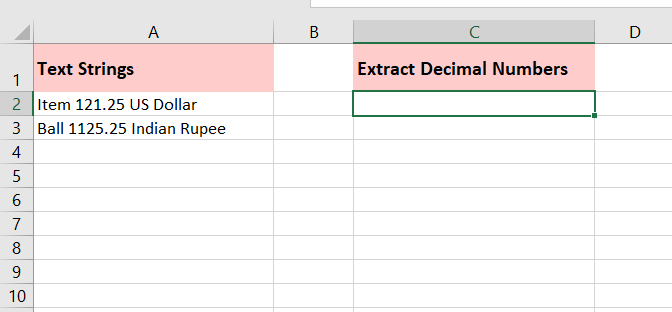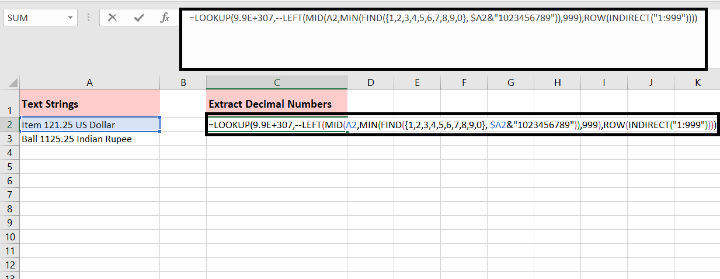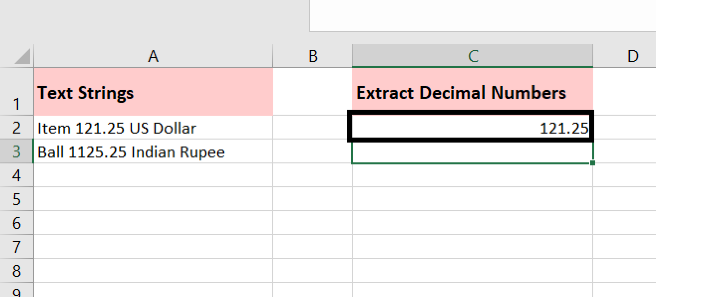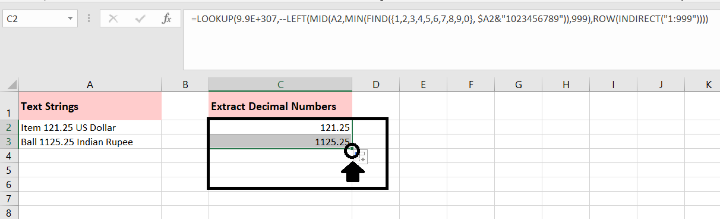# How To Extract Decimal Numbers From Text String In Excel?

Excel is a powerful tool that allows you to perform various calculations and manipulations on data. Sometimes, you may encounter situations where you need to extract decimal numbers from text strings. This can be useful when working with data that includes textual information alongside numeric values. Fortunately, Excel provides several functions that can help you achieve this task efficiently. In this article, we will explore a step−by−step method to extract decimal numbers from text strings in Excel.

Step 1− First, select a blank cell where you want to display the extracted decimal number.Step 2− Enter the following formula in the selected cell−

=LOOKUP(9.9E+307,−−LEFT(MID(A2,MIN(FIND({1,2,3,4,5,6,7,8,9,0}, \$A2&"1023456789")),999),ROW(INDIRECT("1:999"))))Step 3− Press Enter to get the result. The formula will extract the decimal number from cell A2. If you want to extract decimal numbers from a different cell, make sure to adjust the cell reference in the formula accordingly.Step 4− Drag the formula down to apply it to other cells if you have multiple cells from which you want to extract decimal numbers.

To extract decimal numbers from text strings in multiple cells using the formula mentioned, you can follow these steps−

• Step 1− Select the cell C2, which contains the formula for extracting decimal numbers.

• Step 2− Place your cursor on the fill handle (a small square at the bottom−right corner of the selected cell). The cursor will change to a crosshair.

• Step 3− Click and hold the left mouse button, then drag the fill handle down to the cells where you want to apply the formula.

• Step 4− Release the mouse button to fill the formula in the selected cells.

• Step 5− As you drag the fill handle, the formula will adjust the cell references accordingly, extracting decimal numbers from the respective text strings in each cell.Please note that when dragging the fill handle, ensure that you're dragging it over the cells where you want the formula to be applied. The formula will be automatically adjusted for each cell, allowing you to extract decimal numbers from multiple text strings efficiently.

## Conclusion

Extracting decimal numbers from text strings in Excel can be achieved using a combination of functions like MID, SEARCH, and VALUE. By understanding the format of the text strings and leveraging these functions, you can easily extract decimal numbers and use them for various calculations and analysis. With this

Updated on: 13-Jul-2023

441 Views